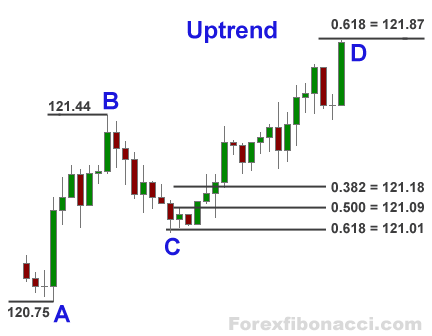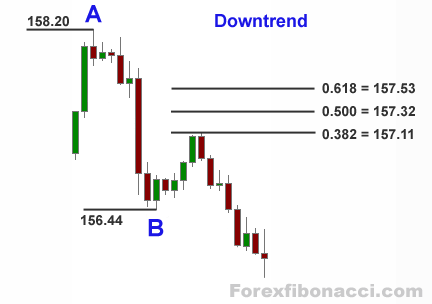# Fibonacci method in Forex

### Forex trading with Fibonacci method 📈 Mini-lesson on how to use FibonacciHOMEFIBONACCI FANCALCULATE FIBONACCI LEVELSFIBONACCI CALCULATOR FIBONACCI FAQ

## How to calculate Fibonacci retracement and extension levels

Three most used Fibonacci retracement levels are 0.382 or 38.2%, 0.500 (50%) and 0.618 (61.8%).

Three most used Fibonacci extension levels are 0.618, 1.000 and 1.618. Also 1.382 extension can be applied as well.

Let's take a look at the next picture:In the example above we are in the uptrend. Lowest swing — point A — is 120.75;
highest swing — point B — 121.44.

To calculate retracement levels and enter Long at some point C we do next:

Calculations for Uptrend and Buy order:

B — A = ?
121.44 — 120.75 = 0.69

0.382 (38.2%) retracement = 121.44 — 0.69 x 0.382 = 121.18
0.500 (50.0%) retracement = 121.44 — 0.69 x 0.500 = 121.09
0.618 (61.8%) retracement = 121.44 — 0.69 x 0.618 = 121.01

Fibonacci retracement levels formula for an uptrend:

C = B — (B — A) x N%

Now we need to calculate extension levels:

0.618 (61.8% ) extension = 121.44 + 0.69 x 0.618 = 121.87
1.000 (100.0%) extension = 121.44 + 0.69 x 1.000 = 122.13
1.382 (138.2%) extension = 121.44 + 0.69 x 1.382 = 122.39
1.618 (161.8%) extension = 121.44 + 0.69 x 1.618 = 122.56

Fibonacci extension levels formula for an uptrend:

D = B + (B — A) x N%

Our next example is downtrend.Highest swing — point A — is 158.20; lowest swing — point B — is 156.44.

Calculations for downtrend and Sell order:

A — B = ?
158.20 — 156.44 = 1.76

Because of the downtrend we need to add to the lowest point B to find retracement.

0.382 (38.2%) retracement = 156.44 + 1.76 x 0.382 = 157.53
0.500 (50.0%) retracement = 156.44 + 1.76 x 0.500 = 157.32
0.618 (61.8%) retracement = 156.44 + 1.76 x 0.618 = 157.11

Fibonacci retracement levels formula for downtrend:

C = B + (A — B) x N%

Now let's find Fibonacci extension levels (downtrend):

0.618 (61.8%) extension = 156.44 — 1.76 x 0.618 = 155.35
1.000 (100%) extension = 156.44 — 1.76 x 1.000 = 154.68
1.382 (138.2%) extension = 156.44 — 1.76 x 1.382 = 154.01
1.618 (161.8%) extension = 156.44 — 1.76 x 1.618 = 153.59

Fibonacci extension levels formula for downtrend:

D = B — (A — B) x N%

Jeff Boyd

## RESOURCES

🔎 Forex brokers

📈 Currency Forecasts

🔑 Free Forex strategiesHOMEFIBONACCI FANCALCULATE FIBONACCI LEVELSFIBONACCI CALCULATOR